# Full and Complete Binary Trees Binary Tree Theorems

• Slides: 8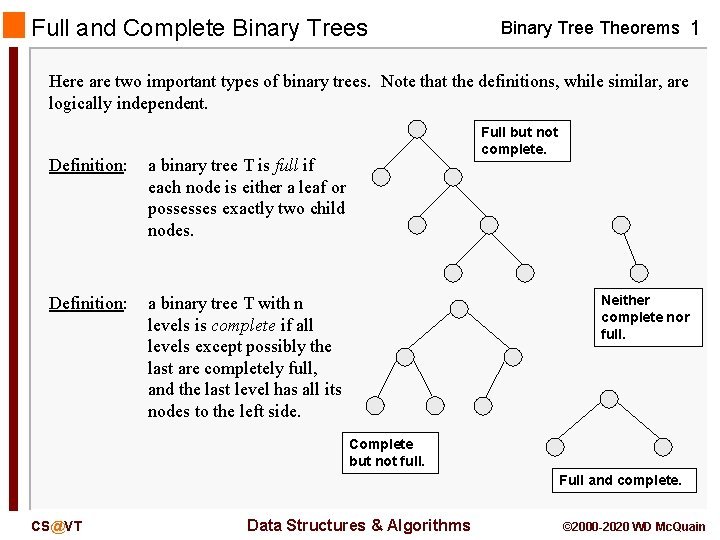Full and Complete Binary Trees Binary Tree Theorems 1 Here are two important types of binary trees. Note that the definitions, while similar, are logically independent. Definition: a binary tree T is full if each node is either a leaf or possesses exactly two child nodes. Definition: a binary tree T with n levels is complete if all levels except possibly the last are completely full, and the last level has all its nodes to the left side. Full but not complete. Neither complete nor full. Complete but not full. Full and complete. [email protected] Data Structures & Algorithms © 2000 -2020 WD Mc. QuainFull Binary Tree Theorem: Binary Tree Theorems 2 Let T be a nonempty, full binary tree. Then: (a) If T has I internal nodes, the number of leaves is L = I + 1. (b) If T has I internal nodes, the total number of nodes is N = 2 I + 1. (c) 1)/2. If T has a total of N nodes, the number of internal nodes is I = (N – (d) If T has a total of N nodes, the number of leaves is L = (N + 1)/2. (e) If T has L leaves, the total number of nodes is N = 2 L – 1. (f) If T has L leaves, the number of internal nodes is I = L – 1. Basically, this theorem says that the number of nodes N, the number of leaves L, and the number of internal nodes I are related in such a way that if you know any one of them, you can determine the other two. [email protected] Data Structures & Algorithms © 2000 -2020 WD Mc. Quain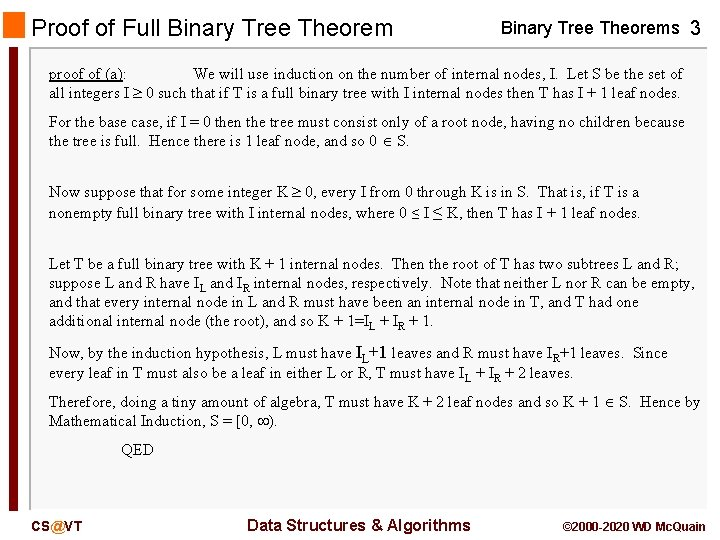Proof of Full Binary Tree Theorems 3 proof of (a): We will use induction on the number of internal nodes, I. Let S be the set of all integers I 0 such that if T is a full binary tree with I internal nodes then T has I + 1 leaf nodes. For the base case, if I = 0 then the tree must consist only of a root node, having no children because the tree is full. Hence there is 1 leaf node, and so 0 S. Now suppose that for some integer K 0, every I from 0 through K is in S. That is, if T is a nonempty full binary tree with I internal nodes, where 0 ≤ I ≤ K, then T has I + 1 leaf nodes. Let T be a full binary tree with K + 1 internal nodes. Then the root of T has two subtrees L and R; suppose L and R have IL and IR internal nodes, respectively. Note that neither L nor R can be empty, and that every internal node in L and R must have been an internal node in T, and T had one additional internal node (the root), and so K + 1=IL + IR + 1. Now, by the induction hypothesis, L must have IL+1 leaves and R must have IR+1 leaves. Since every leaf in T must also be a leaf in either L or R, T must have IL + IR + 2 leaves. Therefore, doing a tiny amount of algebra, T must have K + 2 leaf nodes and so K + 1 S. Hence by Mathematical Induction, S = [0, ). QED [email protected] Data Structures & Algorithms © 2000 -2020 WD Mc. QuainLimit on the Number of Leaves Theorem: Binary Tree Theorems 4 Let T be a binary tree with l levels. Then the number of leaves is at most 2 l -1. proof: We will use strong induction on the number of levels, l. Let S be the set of all integers l 1 such that if T is a binary tree with l levels then T has at most 2 l-1 leaf nodes. For the base case, if l = 1 then the tree must have one node (the root) and it must have no child nodes. Hence there is 1 leaf node (which is 2 l-1 if l = 1), and so 1 S. Now suppose that for some integer K 1, all the integers 1 through K are in S. That is, whenever a binary tree has M levels with M K, it has at most 2 M-1 leaf nodes. Let T be a binary tree with K + 1 levels. If T has the maximum number of leaves, T consists of a root node and two nonempty subtrees, say S 1 and S 2. Let S 1 and S 2 have M 1 and M 2 levels, respectively. Since M 1 and M 2 are between 1 and K, each is in S by the inductive assumption. Hence, the number of leaf nodes in S 1 and S 2 are at most 2 K-1 and 2 K-1, respectively. Since all the leaves of T must be leaves of S 1 or of S 2, the number of leaves in T is at most 2 K-1 + 2 K-1 which is 2 K. Therefore, K + 1 is in S. Hence by Mathematical Induction, S = [1, ). QED [email protected] Data Structures & Algorithms © 2000 -2020 WD Mc. Quain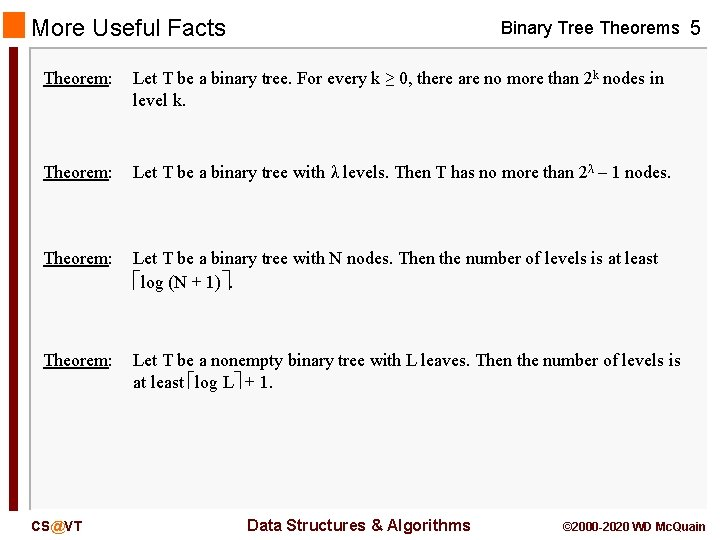More Useful Facts Binary Tree Theorems 5 Theorem: Let T be a binary tree. For every k ≥ 0, there are no more than 2 k nodes in level k. Theorem: Let T be a binary tree with λ levels. Then T has no more than 2λ – 1 nodes. Theorem: Let T be a binary tree with N nodes. Then the number of levels is at least log (N + 1). Theorem: Let T be a nonempty binary tree with L leaves. Then the number of levels is at least log L + 1. [email protected] Data Structures & Algorithms © 2000 -2020 WD Mc. QuainA Note on Terminology Binary Tree Theorems 6 The definition of the height of a tree varies by author. Traditional usage is that the height is the number of levels in the tree. Accordingly, a tree with one node has height 1 and an empty tree has height 0. Some authors now say that the height is the number of edges in the longest path from the root of the tree to a leaf. Accordingly, a tree with one node has height 0 and an empty tree has height ? ? . FWIW, I consider the second usage to be perverse. In this course, we will refer to the number of levels (of nodes) in the tree rather than the height of the tree. [email protected] Data Structures & Algorithms © 2000 -2020 WD Mc. Quain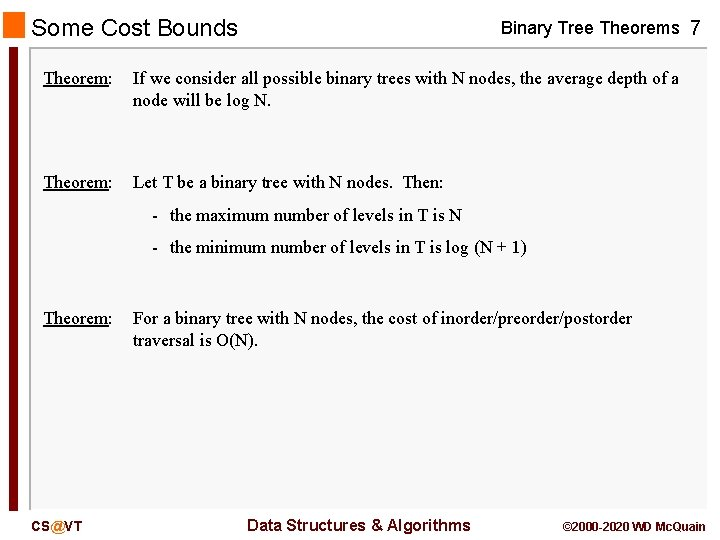Some Cost Bounds Binary Tree Theorems 7 Theorem: If we consider all possible binary trees with N nodes, the average depth of a node will be log N. Theorem: Let T be a binary tree with N nodes. Then: - the maximum number of levels in T is N - the minimum number of levels in T is log (N + 1) Theorem: [email protected] For a binary tree with N nodes, the cost of inorder/preorder/postorder traversal is O(N). Data Structures & Algorithms © 2000 -2020 WD Mc. Quain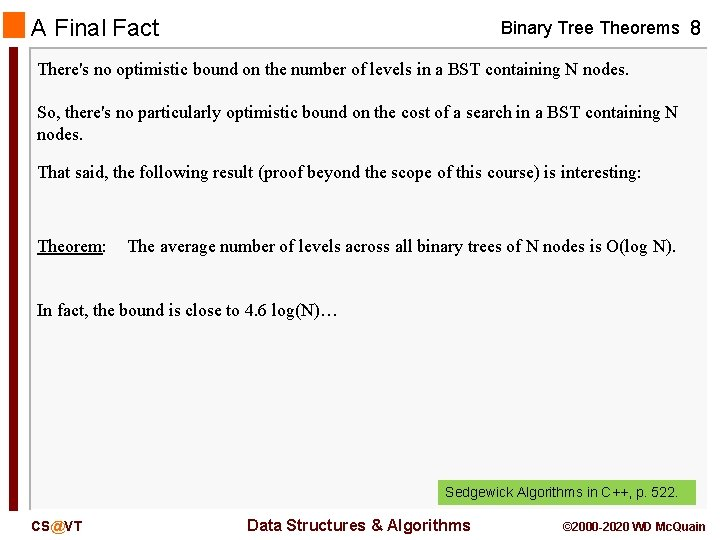A Final Fact Binary Tree Theorems 8 There's no optimistic bound on the number of levels in a BST containing N nodes. So, there's no particularly optimistic bound on the cost of a search in a BST containing N nodes. That said, the following result (proof beyond the scope of this course) is interesting: Theorem: The average number of levels across all binary trees of N nodes is O(log N). In fact, the bound is close to 4. 6 log(N)… Sedgewick Algorithms in C++, p. 522. [email protected] Data Structures & Algorithms © 2000 -2020 WD Mc. Quain